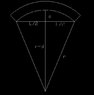# Radius of Curvature Derivation for Bending of Beam w/Overhangs

• Freyster98
L/2 is similar to the triangle with sides L/2 and … ? :smile:In summary, the conversation discusses deriving an expression for the radius of curvature of a beam using geometric methods. The method of using the Pythagorean theorem is suggested, but a quicker method using similar triangles is also mentioned. The original poster also asks for clarification on whether their method is correct.

## Homework Statement

Derive an expression geometrically for the radius of curvature of the following beam. This is part of a lab assignment for the bending of a simply supported beam with overhangs.

** I did this crappy diagram with AutoCAD, so I couldn't ( or didn't know how to ) include greek letters. Let's let r= $$\rho$$, and d= $$\delta$$ for my derivation.

a2+b2=c2

## The Attempt at a Solution

I just used the pythagorean theorem to solve for $$\rho$$.

Starting with: $$\rho$$2= ($$\rho$$-$$\delta$$)2+(L/2)2.

Factoring out ($$\rho$$-$$\delta$$)2 , solving for $$\rho$$ and simplifying , I end up with the following expression:

$$\rho$$=($$\delta$$/2)+(L2/8$$\delta$$)

I guess I have this question...is this the proper way to derive the radius of curvature geometrically? Is it ok to do it this way?

#### Attachments

•11.6 KB · Views: 393
Last edited:
Freyster98 said:
Derive an expression geometrically for the radius of curvature of the following beam.

I just used the pythagorean theorem to solve for $$\rho$$.

Starting with: $$\rho$$2= ($$\rho$$-$$\delta$$)2+(L/2)2.

Factoring out ($$\rho$$-$$\delta$$)2 , solving for $$\rho$$ and simplifying , I end up with the following expression:

$$\rho$$=($$\delta$$/2)+(L2/8$$\delta$$)

I guess I have this question...is this the proper way to derive the radius of curvature geometrically? Is it ok to do it this way?

Hi Freyster98!(have a rho: ρ and a delta: δ)

Yes, Pythagoras is fine(though you seem to have lost a factor of 2 somewhere).

But there is quicker method (with less likelihood of a mistake):

Hint: similar trianglestiny-tim said:
Hi Freyster98!(have a rho: ρ and a delta: δ)

Yes, Pythagoras is fine(though you seem to have lost a factor of 2 somewhere).

But there is quicker method (with less likelihood of a mistake):

Hint: similar trianglesI ran through it a few times...I don't see where I'm losing a factor of 2.

sorry … my similar triangles method (have you tried that yet?) gave me the diameter, not the radiusso i got an extra 2tiny-tim said:
sorry … my similar triangles method (have you tried that yet?) gave me the diameter, not the radiusso i got an extra 2Ok, thanks. No, I haven't tried the similar triangles because, well, I don't get it :uhh:

Freyster98 said:
Ok, thanks. No, I haven't tried the similar triangles because, well, I don't get it :uhh:

ok … the triangle with sides d and L/2 is similar to the triangle with sides L/2 and … ?